Vibration Control Using Piezoelectric Transducers

J. Bert October 29, 2007

(Submitted as coursework for Physics 210, Stanford University, Fall 2007)

Controlling mechanical motion and damping unwanted vibrations in flexible structures can be achieved using piezoelectric transducers (piezos) . Piezoelectric materials are crystals or ceramics that generate an internal voltage when stressed. If a piezoelelectric element is rigidly mounted to a flexible structure, then vibrations and deformations in the structure are coupled to the attached piezoelectric transducer. Using the voltage induced in the piezo as an input signal the stress may be monitored or controlled using an external shunt or feedback circuit.

This paper briefly describes the physical origins of the piezoelectric effect and then details how piezoelectric sensors and actuators are used in vibration control devices. Vibration control is achieved by using the signal generated from the piezo element as the input signal to an external circuit. The circuit can either apply feedback by processing the input piezo voltage and applying the output signal back to the piezo element, or the circuit can simply dissipate vibrational energy by electrically damping the piezo element.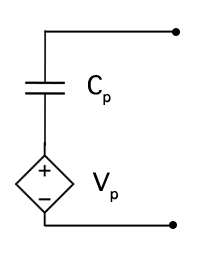Figure 1: Piezoelectric element equivalent circuit.

The Piezoelectric Effect

Piezoelectric materials are typically ceramic or crystalline in structure, with permanently aligned electric dipoles. The dipoles allow for separation of positive and negative charges within the material, but the symmetry of the crystal assures that there is no internal electric field. However, if the crystal is stressed then the crystal symmetry is broken and an internal electric field is generated between surfaces of the piezo. If the internal field is not compensated, for example by shorting one side of the piezo to the other, it results in an induced voltage. The effect of stressing a piezo to generate a voltage is called the direct piezo effect. The converse piezo effect involves inducing a stress in the piezo element by a apply an external electric field or potential.

Piezos are fabricated by adding electrodes to the surfaces of the ceramic or crystal to either probe the stress induced voltage or apply a local voltage to the element. An external field applied parallel to the alignment direction of the dipoles within the piezo material will cause the piezo to expand along the field direction and contract in the two directions perpendicular to the field.

The external electrodes and the charge arrangement within the piezo material give the piezo element a capacitance, Cp. As a result the equivalent circuit for a piezo element is the capacitance, Cp, and internal voltage, Vp arranged in series as shown in figure 1.

Sensors and Actuators

To use a piezo as either a sensor or actuator for a vibration control device it must be rigidly mounted to the vibration sensitive part. This assures that any stress or strain in the static structure is rigidly coupled to the piezo and vice versa. Piezos used as sensors are often light and flexible to provide the best transmission of the mechanical vibration into the piezo for detection. Piezos used as actuators are often denser so that the stress induced in the piezo by an applied voltage is large enough to stress the structure as well.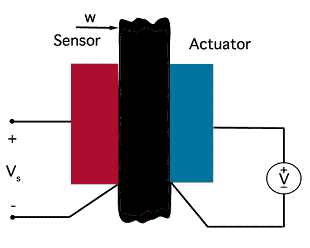Figure 2: Flexible structure with collocated sensor and actuator piezos.

One of the simplest vibration control circuits is a collocated pair of sensor/actuator piezo elements. Collocated means that the two piezos are placed in the same position on two different sides of a flexible structure, as shown in figure 2. Assuming the two piezos are identical, meaning they have the same internal capacitance, if the structure flexes the voltages induced in the two piezos will be equal but 180º out of phase. After correctly resolving the transfer functions between the vibrating structure and the voltage generated in the sensor and between the voltage measured by the sensor and the voltage applied to the actuator, feedback control can be established between the sensor output and actuator to oppose the vibration. This simple model is easy to imagine implementing; however, in practice small errors in the transfer functions can quickly destabilize the feedback.

Self-Sensing Piezos

A more stable, although more complicated, feedback system can be set up with a self sensing piezo actuator . Such a setup requires just one piezo to be mounted to the structure. The strain applied by the structure to the piezo generates a voltage, Vp, which rather than being fed back to a separate piezo can be used to compensate the same piezo that generated the signal. The single piezo acts as both sensor and actuator in the circuit.

One complication for this scheme is that the induced voltage cannot be probed directly. The external circuit shown in figure 3 is required to separate Vp from the internal capacitor voltage, Q/Cp, and deduce the strain. The strain voltage is fed back into the input of the same piezo to compensate the mechanical vibration.

Assuming the leakage resistors R1 and R2 are very large and the operational amplifiers have a gain of one, leads to the following expressions for the output voltage.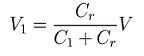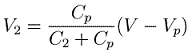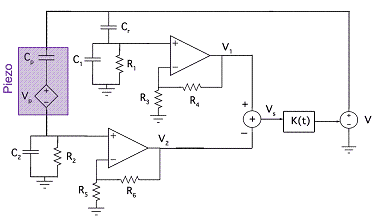Figure 3: Feedback circuit for a self-sensing damping piezo. K(t) is an additional feedback control function which for this discussion is taken to transmit Vs directly back to V. The piezo is represented by its equivalent circuit shown in the purple box.

Here Vp is the voltage induced in the piezo and Cp is the capacitance of the piezo. This circuit tries to recreate the effect of the capacitance of the piezo in the first branch and then subtracts the recreated signal from the signal generated by the piezo to find the induced voltage. The key to this design is matching the external capacitor Cr with the internal capacitance of the piezo, Cp. If indeed Cr is chosen so that Cr=Cp then subtracting V1 and V2 from equations 1 and 2 yields: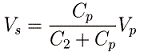The differential voltage, Vs is directly proportional to the voltage induced in the piezo.

Similar external circuits have also been designed to calculate the strain rate, dVp/dt , yet most of these methods rely on accurately matching the internal capacitance of the piezo. In practice this is very difficult to achieve, mainly because Cp is highly dependent on the environment and operating conditions. For example, operating the piezo in an external field may drastically change it's intrinsic behavior. In any situation where a feedback circuit is operated in a closed loop, meaning the output is fed back to the input, small errors in the output signal may excite rather than damp the system and can quickly snowball and destabilize it. Either extremely accurate knowledge of the internal capacitance or a dynamic adaptive feedback circuit is required for successful operation of closed loop feedback.

Shunted Piezos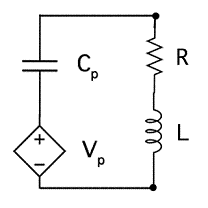Figure 4: RL shunted piezo.

A third scheme first proposed by R. L. Forward involves using external electric shunt circuits connected to the piezo to quickly damp mechanical vibrations in the structure . Shunting the piezo is a passive technique, so it avoids the problem of having destabilizing errors build up in a feedback loop. The piezo changes the mechanical energy into electrical energy which diverted into a resonant LC circuit and electrically dissipated. An common shunt circuit is shown in figure 4, which includes an external resistor in addition the LC resonator formed by the internal capacitance of the piezo, Cp and an external inductor, L . The inductor is selected to damp a specific vibrational mode with frequency ωi.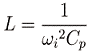The same problem of knowing the internal capacitance of the piezo, Cp comes up again; however, in this case an error results in a slightly different vibrational mode being damped rather than a destabilization of the circuit. The main drawback to using an RL shunt is that the shunt is only able to damp one mode. To introduce further vibration control one must add more passive elements. One possible technique, proposed by Wu et al. uses a parallel RL shunt for each damped mode wired in series with a blocking LC circuit[5-6]. The circuit diagram for two mode damping is shown in figure 5.

In a parallel LC circuit the inductor passes low frequencies and the capacitor passes high frequencies, only the resonance frequency of the circuit, ω=1/(LC)1/2, will be blocked by the filter. To damp two modes in the base structure, ω1 and ω2, L1Cp is tuned to ω1 and L2Cp is tuned to ω2, these circuits will directly shunt the respective modes. In the blocking circuits, L1Cp* is tuned to ω2 while L2Cp* is tuned to ω1. This prevents the shunts from being exposed to modes that are damped elsewhere in the circuit.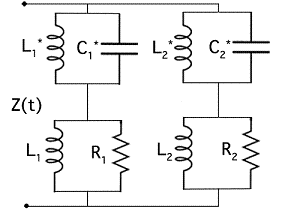Figure 5: Equivalent impedance for two mode shunt damping circuit.

Wu's design is an elegant way to add more modes without retuning each previous branch, but the method does not scale well. For every mode that is shunted with an RL circuit that mode must be blocked on all other branches. To damp three modes requires six blocking LC circuits in addition to the three RL shunts, requiring 2n2 passive elements to damp n modes. To complicate things even further damping very low frequencies requires fairly large inductors on the order of tens of henrys. These inductors are often replaced by gyrator circuits that use an operational amplifier, two resistors and a capacitor to accurately simulate an inductor. Gyrator circuits are often cheaper, less bulky and more reliable than very large inductors. If gyrator circuits are used to replace the inductors then 4n2-2n operational amplifiers are required to damp n modes. Operational amplifiers are active rather than passive elements and require external power. Replacing inductors with gyrator circuits is often necessary, but it makes scaling the circuit to damp more modes even more difficult.

Many other passive shunt circuits have been designed but all suffer from the same scaling problem [7-8]. They work well if the piezo element is used to damp a small number of vibrational modes; however, they do not scale well and therefore cannot be used to damp multiple vibrational modes. To address a large number of vibrational modes other solutions must be sought.

Shunted Feedback Circuits

This paper has covered using a post-processed signal to feedback and control the motion of the piezo and applying external passive shunt to electronically damp vibrations of the piezo. Both have been shown to exhibit debilitating problems, especially when applied to a large number of modes. A third solution combines these two methods by using feedback to digitally generate an impedance which dynamically shunts the piezo. A dynamic impedance eliminates the problem of having to address all vibrational modes individually, and feeding back on an external impedance circuit rather than the piezo itself is more stable. A schematic of a feedback circuit proposed and implemented by Moheimani et. al is shown in figure 6B . In this implementation the admittance Y(t) is used as the control for the circuit. Gvv(t) is the transfer functions that determines how voltage applied to the piezo transfers to motion in the structure is related to the voltage the piezo senses, and the transfer function Gvw(t) determines how a vibration in the structure, w, is related to the induced voltage. Both of these transfer functions affect the total induced voltage in the piezo, Vp(t). Cp is the capacitance of the piezo and V(t) is the voltage dropped across the admittance element. The following expressions describe the behavior of the circuit in figure 6A.

Two individual feedback loops within the circuit feed back using the voltage induced in the piezo and the voltage across the admittance. The challenge of designing this feedback circuit is determining an algorithm for the admittance. Many possible solutions exists and each must be optimized to the particular piezo/structure coupling to achieve peak performance. The admittance suggested by Moheimani et. al has been shown to reduce mechanical resonances by 20 dB .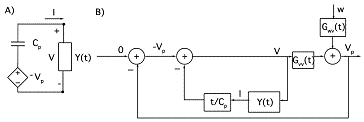Figure 6: A) Schematic of a piezo feedback circuit where the control is the admittance of the shunt circuit. B) Lump diagram of the feedback loop assuming the admittance, Y(t) is controlled.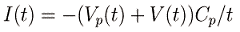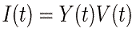Summary

This overview of piezoelectric elements used in vibration damping circuits has covered three basic damping designs including self sensing feedback, passive shunts, and shunted feedback. The brevity and introductory nature of this paper cannot due full justice to the technical complexity as well as the variety of work done in this field. The interested reader is encouraged to refer to the references within this paper to gain a more comprehensive view of the subject.

© 2007 J. Bert. The author grants permission to copy, distribute and display this work in unaltered form, with attribution to the author, for noncommercial purposes only. All other rights, including commercial rights, are reserved to the author.

References

 S. O. R. Moheimani, IEEE TCST 11, 482 (2003).

 J. J. Dosch, D. J. Inman, and E. Garcia, J. Intel. Mat. System and Structures 3, 166 (1992).

 R. L. Forward, J. Appl. Opt. 18, 690 (1979).

 N. W. Hagood and A. von Flotow, J. Sound Vibr. 146, 243 (1991).

 S. Y. Wu, US Patent 5 783 898 (1998).

 S. Y. Wu, Proc. SPIE Symp. Smart Strctures Materials Passive Damping Isolation 3327, 159 (1998).

 J. J. Hollkamp, J. Intel. Mat. System and Structures 5, 49 (1994).

 S. Behens and S. O. R. Moheimani, Proc. SPIE Symp. Smart Strctures Materials Damping Isolation 4697, 217 (2002).

 S. O. R. Moheimani, A. J. Fleming, and S. Behens, Electron. Lett. 18, 442 (2001).